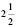# User ForumSubject :IMO    Class : Class 7

A businessman borrowed some money at 1% per month. After some time, he settled the debt by paying 14,750. If the total interest was 2950, find the time.
(a) 2 years 1 month
(b) 3 years
(c)years
(d) 1 year 8 months

explain

## Ans 1:

Class : Class 8
P=A-I =14750-2950 =11800 R= 1% PER MONTH = 12%PER YEAR T=(I*100)/(P*R) (2950*100)/(11800*12) =25/12 = 2 1/12 YEARS = 2 YEARS 1 MONTH